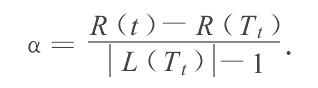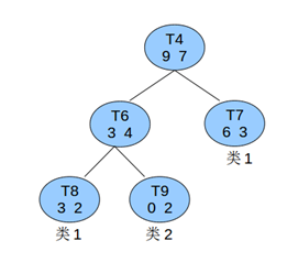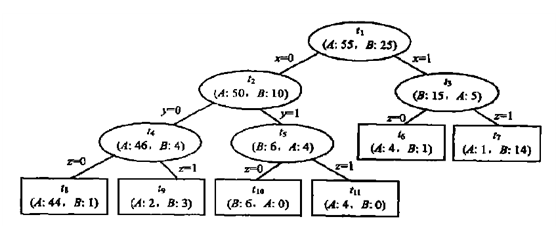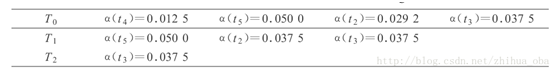# 1. KDTREE

kd树（k-dimensional树的简称），是一种分割k维数据空间的数据结构。主要应用于多维空间关键数据的搜索（如：范围搜索和最近邻搜索）。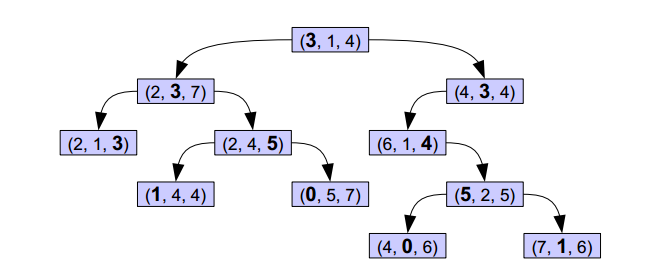## 1.1 从二叉搜索树谈起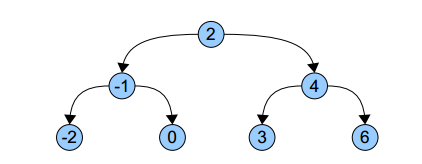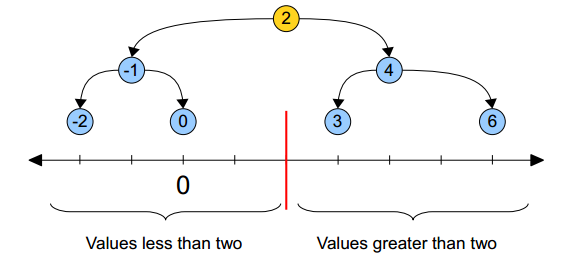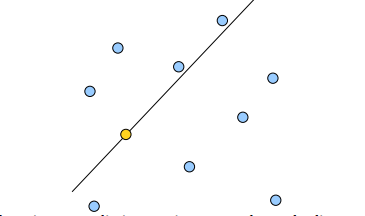KDTree就是超平面都垂直于轴的BSPTree。同样的数据集，用KDTree划分之后就是这样：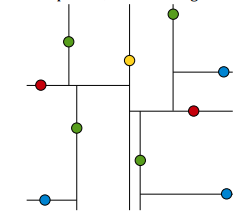1.树的建立；
2.最近邻域搜索（Nearest-Neighbor Lookup）。

## 1.2 树的建立

Node-data - 数据矢量， 数据集中某个数据点，是n维矢量（这里也就是k维）
Range - 空间矢量， 该节点所代表的空间范围
split - 整数， 垂直于分割超平面的方向轴序号
Left - k-d树， 由位于该节点分割超平面左子空间内所有数据点所构成的k-d树
Right - k-d树， 由位于该节点分割超平面右子空间内所有数据点所构成的k-d树
parent - k-d树， 父节点

1.建立的树应当尽量平衡，树越平衡代表着分割得越平均，搜索的时间也就是越少。
2.最大化邻域搜索的剪枝机会。

## 1.3 最近邻搜索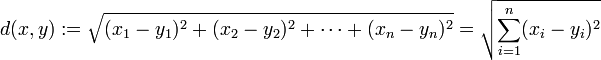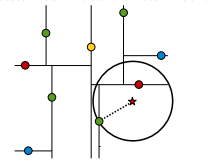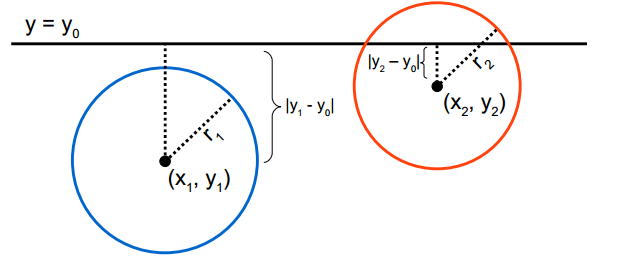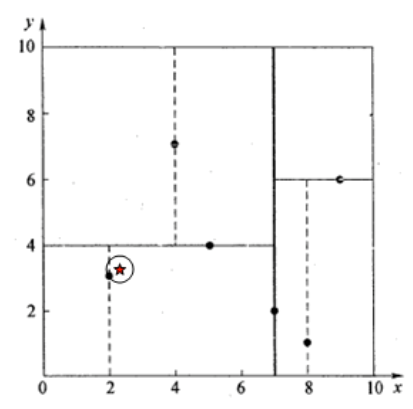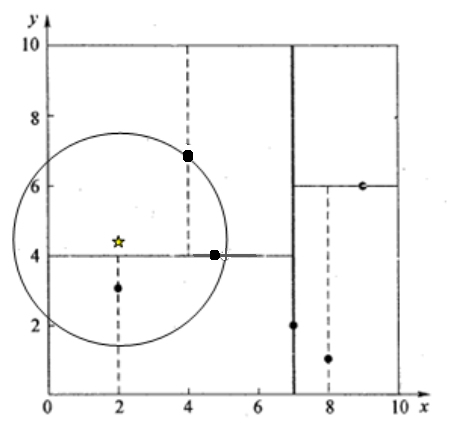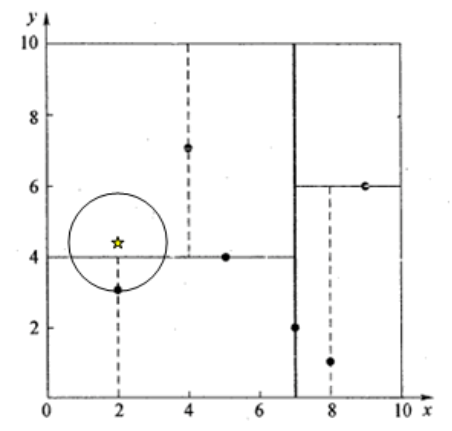# 2. 决策树

## 2.1 熵与条件熵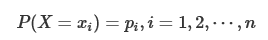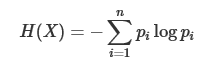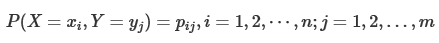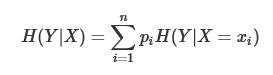(1)计算数据集D 的经验熵H(D)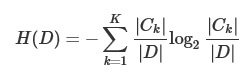(2)计算特征A 对数据集D 的经验条件熵H(D|A)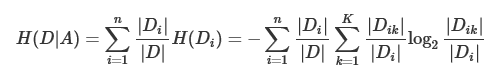(3)计算信息增益
g(D,A)=H(D)−H(D|A)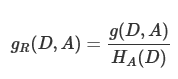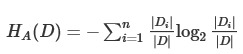n 是特征A 取值的个数。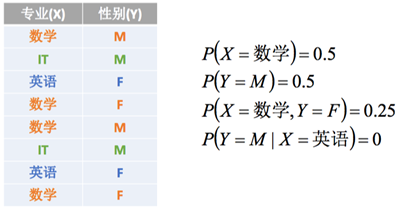H(X) =L(X=数学) + L(X=IT) + L(X=英语)
= -0.5 * log2(0.5) - 0.25 * log2(0.25) - 0.25*log2(0.25)
= 0.5 + 0.5 + 0.5 = 1.5

H(Y) = L(Y = M) + L(Y = F)
= -0.5 * log2(0.5) - 0.5 * log2(0.5)
= 0.5 + 0.5 = 1

H(X, Y) = L(X=数学, Y=M) + L(X=IT, Y=M) + L(X=英语, Y=F) + L(X=数学, Y=F)
= -0.25 * log2(0.25) * 4
= 2

H(X/Y) = H(X/Y=M)*P(Y=M) + H(X/Y=F)*P(Y=F)即加权平均熵
= [L(X=数学/Y=M)+L(X=数学/Y=M)]*P(Y=M) + H(X/Y=F)*P(Y=F)
= [-0.5 * log(0.5) * 2] * 0.5 + H(X/Y=F)*P(Y=F)
= 0.5 + H(X/Y=F)*P(Y=F)= 0.5 + 0.5 = 1
= H(X, Y) - H(Y)

H(Y/X) = H(Y/X=数学)*P(X=数学)+H(Y/X=IT)*P(X=IT)+H(Y/X=英语)*P(X=英语)
= [-0.5 * log(0.5) * 2] * 0.5+H(Y/X=IT)*P(X=IT)+H(Y/X=英语)*P(X=英语)
= 0.5 + 0 + 0 = 0.5
= H(X, Y) - H(X)

## 2.2 ID3（只能分类）

ID3算法由Ross Quinlan发明，建立在“奥卡姆剃刀”的基础上：越是小型的决策树越优于大的决策树（be simple简单理论）。ID3算法中根据信息增益评估和选择特征，每次选择信息增益最大的特征作为判断模块建立子结点。ID3算法可用于划分标称型数据集，没有剪枝的过程，为了去除过度数据匹配的问题，可通过裁剪合并相邻的无法产生大量信息增益的叶子节点（例如设置信息增益阀值）。使用信息增益的话其实是有一个缺点，那就是它偏向于具有大量值的属性。就是说在训练集中，某个属性所取的不同值的个数越多，那么越有可能拿它来作为分裂属性，而这样做有时候是没有意义的，另外ID3不能处理连续分布的数据特征，于是就有了C4.5算法。CART算法也支持连续分布的数据特征。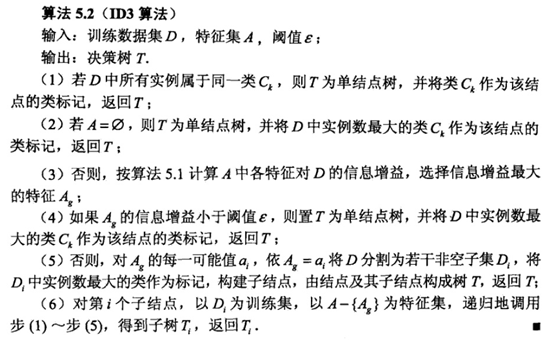## 2.3 ID4.5（只能分类）

C4.5算法用信息增益率来选择属性，继承了ID3算法的优点。并在以下几方面对ID3算法进行了改进：
• 克服了用信息增益选择属性时偏向选择取值多的属性的不足；
• 在树构造过程中进行剪枝；
• 能够完成对连续属性的离散化处理；
• 能够对不完整数据进行处理。

ID3和C4.5二者都有贪心性质：即通过局部最优构造全局最优。C4.5算法产生的分类规则易于理解、准确率较高；但效率低，因树构造过程中，需要对数据集进行多次的顺序扫描和排序。也是因为必须多次数据集扫描，C4.5只适合于能够驻留于内存的数据集。对于离散型描述属性，C4.5的处理方法与ID3相同，按照该属性本身的取值个数进行计算；对于某个连续性描述属性Ac，假设在某个结点上的数据集的样本数量为total，该算法采用如下步骤：

• 将该结点上的所有数据样本按照连续型描述属性的具体数值，由小到大进行排序，得到属性值的取值序列{A1c，A2c，……Atotalc}。
• 在取值序列中生成total-1个分割点。第i（0<i<total）个分割点的取值设置为Vi=（Aic+Ai+1c）/2,它可以将该节点上的数据集划分为两个子集。
• 从total-1个分割点中选择最佳分割点。对于每一个分割点划分数据集的方式，计算它的信息增益比，并且从中选择信息增益比最大的分割点来划分数据集。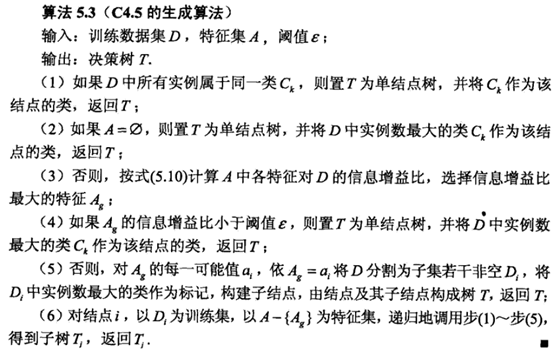## 2.4 CART（能分类，也能回归）

CART，即分类与回归树（classification and regression tree），也是一种应用很广泛的决策树学习方法。但是CART算法比较强大，既可用作分类树，也可以用作回归树。作为分类树时，其本质与ID3、C4.5并有多大区别，只是选择特征的依据不同而已。另外，CART算法建立的决策树一般是二叉树，即特征值只有yes or no的情况。当CART用作回归树时，以最小平方误差作为划分样本的依据。

### 2.4.1 分类树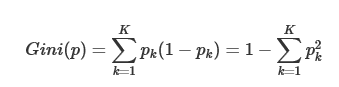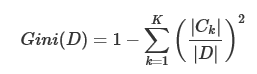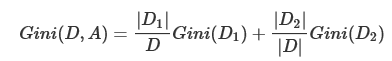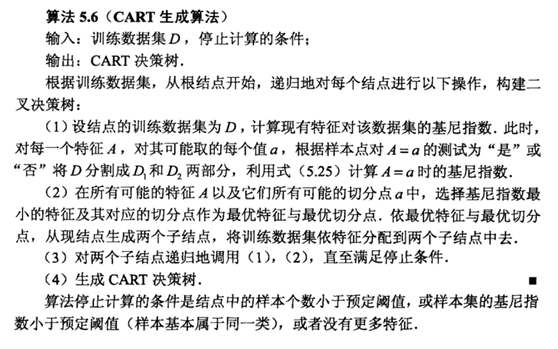### 2.4.1 回归树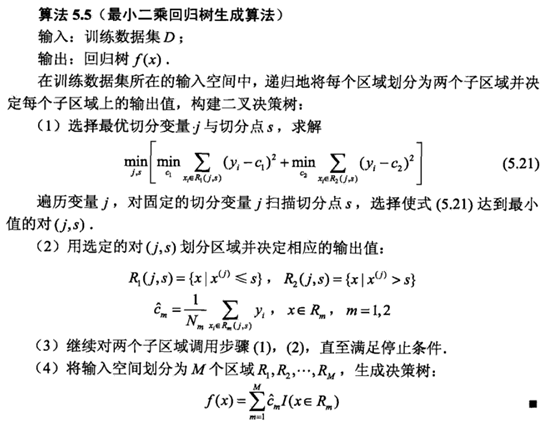## 2.4 剪枝